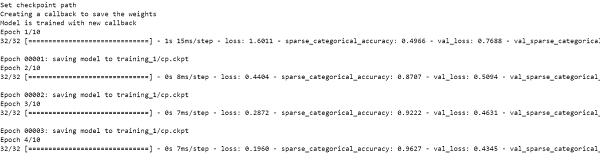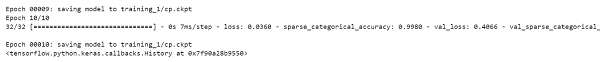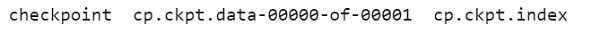# How can Keras be used to create a callback and save the weights using Python?

Tensorflow is a machine learning framework that is provided by Google. It is an open−source framework used in conjunction with Python to implement algorithms, deep learning applications and much more. It is used in research and for production purposes. It has optimization techniques that help in performing complicated mathematical operations quickly. This is because it uses NumPy and multi−dimensional arrays. These multi−dimensional arrays are also known as ‘tensors’.

The ‘tensorflow’ package can be installed on Windows using the below line of code −

pip install tensorflow

Tensor is a data structure used in TensorFlow. It helps connect edges in a flow diagram. This flow diagram is known as the ‘Data flow graph’. Tensors are nothing but multidimensional array or a list.

Keras was developed as a part of research for the project ONEIROS (Open ended Neuro−Electronic Intelligent Robot Operating System). Keras is a deep learning API, which is written in Python. It is a high-level API that has a productive interface that helps solve machine learning problems. It runs on top of Tensorflow framework. It was built to help experiment in a quick manner. It provides essential abstractions and building blocks that are essential in developing and encapsulating machine learning solutions.

Keras is already present within the Tensorflow package. It can be accessed using the below line of code.

import tensorflow
from tensorflow import keras

We are using the Google Colaboratory to run the below code. Google Colab or Colaboratory helps run Python code over the browser and requires zero configuration and free access to GPUs (Graphical Processing Units). Colaboratory has been built on top of Jupyter Notebook. Following is the code −

## Example

print("Set checkpoint path")
checkpoint_path = "training_1/cp.ckpt"
checkpoint_dir = os.path.dirname(checkpoint_path)

print("Creating a callback to save the weights")
cp_callback = tf.keras.callbacks.ModelCheckpoint(filepath=checkpoint_path, save_weights_only=True, verbose=1)

print("Model is trained with new callback")
model.fit(train_images,
train_labels,
epochs=10,
validation_data=(test_images, test_labels),
callbacks=[cp_callback])
ls {checkpoint_dir}

## Output## Explanation

• The trained model can be used without re-training it or training it from the point it was left off.

• The ‘ModelCheckpoint’ method continuously saves the model during and at the end of the training.

• This way, checkpoint files are updated after every epoch.

• This model is fit to the training data.